## KNN using c++ for nRF51822, any suggestion for this output?

Hello world. After I have used this guy code.. KNN c++. and good for implement it to my module nRF51822. But if there's problem issue or not. because, there was an confusing problem. that show like this one:

1. if I'm using this

#### include the mbed library with this snippet

``` // Sort the Points by distance from p
sort(arr, arr+n, comparison);
```

#### Quote:

error: Identifier "sort" is undefined "sort (arr, arr+n, comparison);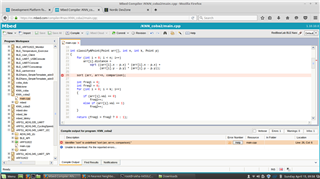but if I clear that code, to be comment session. that's compile without problem..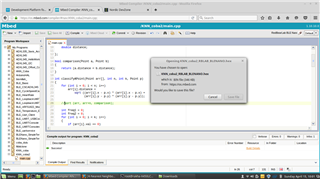and the output data that's look like this one..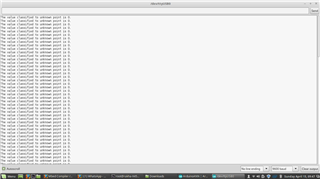but after several browsing from another forum, I get this one solution.. using this function

1. include <algorithm> and std::sort that can active the sorting function..

here's the code..

#### include the mbed library with this snippet

```#include "mbed.h"
#include "Serial.h"
#include <algorithm>
Serial uart1(USBTX,USBRX);
using namespace std;
...

nt classifyAPoint(Point arr[], int n, int k, Point p)
{
for (int i = 0; i < n; i++)
arr[i].distance =
sqrt ((arr[i].x - p.x) * (arr[i].x - p.x) +
(arr[i].y - p.y) * (arr[i].y - p.y));

std::sort (arr, arr+n, comparison);
...
}
```

any suggestion, what supposed I do for the next step? this is my mbed code1 thanks, if your kindness, please give me the link code for the proper KNN that can classify data..

2. and there's also, I wanna ask too. why this code don't have same output value from this one..KNN c++ 2

#### include the mbed library with this snippet

```#include<stdio.h>
#include "mbed.h"
#include "Serial.h"
Serial uart1(USBTX,USBRX);
/* Function to find the cross over point (the point before
which elements are smaller than or equal to x and after
which greater than x)*/
int findCrossOver(int arr[], int low, int high, int x)
{
// Base cases
if (arr[high] <= x) // x is greater than all
return high;
if (arr[low] > x)  // x is smaller than all
return low;

// Find the middle point
int mid = (low + high)/2;  /* low + (high - low)/2 */

/* If x is same as middle element, then return mid */
if (arr[mid] <= x && arr[mid+1] > x)
return mid;

/* If x is greater than arr[mid], then either arr[mid + 1]
is ceiling of x or ceiling lies in arr[mid+1...high] */
if(arr[mid] < x)
return findCrossOver(arr, mid+1, high, x);

return findCrossOver(arr, low, mid - 1, x);
}

// This function prints k closest elements to x in arr[].
// n is the number of elements in arr[]
void printKclosest(int arr[], int x, int k, int n)
{
// Find the crossover point
int l = findCrossOver(arr, 0, n-1, x);
int r = l+1;   // Right index to search
int count = 0; // To keep track of count of elements already printed

// If x is present in arr[], then reduce left index
// Assumption: all elements in arr[] are distinct
if (arr[l] == x) l--;

// Compare elements on left and right of crossover
// point to find the k closest elements
while (l >= 0 && r < n && count < k)
{
if (x - arr[l] < arr[r] - x)
uart1.printf("%d ", arr[l--]);
else
uart1.printf("%d ", arr[r++]);
count++;
}

// If there are no more elements on right side, then
// print left elements
while (count < k && l >= 0)
uart1.printf("%d ", arr[l--]), count++;

// If there are no more elements on left side, then
// print right elements
while (count < k && r < n)
uart1.printf("%d ", arr[r++]), count++;
}

/* Driver program to check above functions */
int main()
{
uart1.baud(9600);
wait(0.1);
int arr[] ={12, 16, 22, 30, 35, 39, 42,
45, 48, 50, 53, 55, 56};
int n = sizeof(arr)/sizeof(arr);
int x = 35, k = 4;
printKclosest(arr, x, 4, n);
while(1)
{
wait(0.1);
uart1.printf("%d \n",printKclosest);
}
//return 0;
}
```

the output shows must be :

#### Quote:

39 30 42 45

but my code just around this one..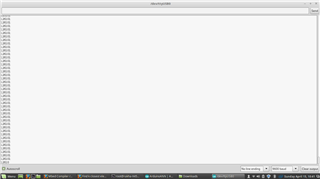3. this is I compiled it from this guy KNN c++ 3 here's my code to mbed...

#### include the mbed library with this snippet

```#include "mbed.h"
#include <iostream>
#include "conio.h"
#include "Serial.h"
Serial uart1(USBTX,USBRX);

int main()
{
uart1.baud(9600);
wait(0.1);
iint i,j,l;
int tsc=12;
char gen={'F','M','F','F','F','M','F','M','M','F','M','F'};
float h={1.6f,2.0f,1.9f,1.88f,1.7f,1.85f,1.6f,1.7f,2.2f,1.8f,1.95f,1.9f};
char op={"short","tall","medium","medium","short","medium","short","short","tall","medium","medium","medium"};

uart1.printf("\n Initial Set:");
uart1.printf("\nGender\tHeight\tOutput");
for(i=0;i<12;i++)
{
uart1.printf("%d, %d, %d \n",gen[i], h[i], op[i]);
}

float nh;
char ng;
uart1.printf("\n Enter tuple to be processed (Height,Gender) :");
cin>>nh>>ng;

int t;
uart1.printf("\n Enter threshold:");
cin>>t;

float d,k;

//calculating distance to each value in training set
for(i=0;i<12;i++)
{
d[i]=i;
k=h[i]-nh;
if(k<0)
{
d[i]=-k;
}
else
{
d[i]=k;
}
}

//Sorting
for(i=0;i<11;i++)
{
for(j=0;j<11;j++)
{
if(d[j]>d[j+1])
{
k=d[j];
d[j]=d[j+1];
d[j+1]=k;

l=d[j];
d[j]=d[j+1];
d[j+1]=l;
}
}
}

int nos=0;  //no of shorts
int nom=0;  //no of mediums
int not=0;  //no of talls

uart1.printf("\nGender\tHeight\tOutput\n");
for(i=0;i<t;i++)
{
l=d[i];
uart1.printf("%d, %d, %d\n",gen[l],h[l],op[l]);
if(strcmp(op[l],"short")==0)
{
nos++;
}
if(strcmp(op[l],"medium")==0)
{
nom++;
}
if(strcmp(op[l],"tall")==0)
{
not++;
}
}

uart1.printf("\n No of shorts:")<<nos;
uart1.printf("\n No of medium:")<<nom;
uart1.printf("\n No of tall:")<<not;

if(nos>nom&&nos>not)
{
uart1.printf("\n New Tuple is classified as Short");
}

if(nom>nos&&nom>not)
{
uart1.printf("\n New Tuple is classified as Medium");
}

if(not>nom&&not>nos)
{
uart1.printf("\n New Tuple is classified as Tall");
}

getch();
}
```

but there was error message like this one.

#### Quote:

Error: #error directive: "CMSIS Target not recognised" in "extras/mbed/cmsis.h", Line: 16, Col: 3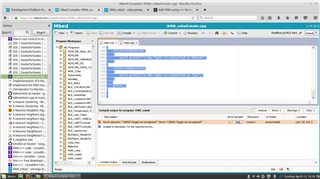please solve this soon..

Be the first to answer this question.